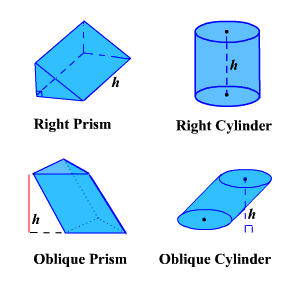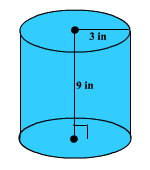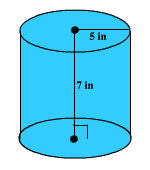# Surface Area of a Cylinder

Since a cylinder is closely related to a prism , the formulas for their surface areas are related.Remember the formulas for the lateral surface area of a prism is $ph$ and the total surface area is $ph+2B$ .  Since the base of a cylinder is a circle, we substitute $2\pi r$ for $p$ and $\pi {r}^{2}$ for $B$ where $r$ is the radius of the base of the cylinder.

So, the formula for the lateral surface area of a cylinder is $L.S.A.=2\pi rh$ .

Example 1:

Find the lateral surface area of a cylinder with a base radius of $3$ inches and a height of $9$ inches.$L.S.A.=2\pi \left(3\right)\left(9\right)=54\pi \text{\hspace{0.17em}}{\text{inches}}^{2}$

$\approx 169.64\text{\hspace{0.17em}}{\text{inches}}^{2}$

The general formula for the total surface area of a cylinder is $T.S.A.=2\pi rh+2\pi {r}^{2}$ .

Example 2:

Find the total surface area of a cylinder with a base radius of $5$ inches and a height of $7$ inches.$\begin{array}{l}T.S.A.=2\pi \left(5\right)\left(7\right)+2\pi {\left(5\right)}^{2}\\ =120\pi \text{\hspace{0.17em}}{\text{inches}}^{2}\\ \approx 376.99\text{\hspace{0.17em}}{\text{inches}}^{2}\end{array}$